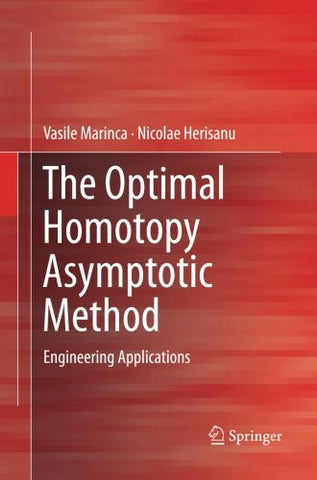Shopping Cart### The Optimal Homotopy Asymptotic Method: Engineering Applications

Regular price \$239.98 Sale price

Compare at \$290.24

• ISBN-13: 9783319361833
• Publisher: Springer
• Release Date: Nov 18, 2016
• Edition: Softcover reprint of the original 1st ed. 2015
• Pages: 465 pages
• Dimensions: 1.08 x 9.25 x 6.1 inches

### Overview

This book emphasizes in detail the applicability of the Optimal Homotopy Asymptotic Method to various engineering problems. It is a continuation of the book “Nonlinear Dynamical Systems in Engineering: Some Approximate Approaches”, published at Springer in 2011 and it contains a great amount of practical models from various fields of engineering such as classical and fluid mechanics, thermodynamics, nonlinear oscillations, electrical machines and so on. The main structure of the book consists of 5 chapters. The first chapter is introductory while the second chapter is devoted to a short history of the development of homotopy methods, including the basic ideas of the Optimal Homotopy Asymptotic Method. The last three chapters, from Chapter 3 to Chapter 5, are introducing three distinct alternatives of the Optimal Homotopy Asymptotic Method with illustrative applications to nonlinear dynamical systems. The third chapter deals with the first alternative of our approach with two iterations. Five applications are presented from fluid mechanics and nonlinear oscillations. The Chapter 4 presents the Optimal Homotopy Asymptotic Method with a single iteration and solving the linear equation on the first approximation. Here are treated 32 models from different fields of engineering such as fluid mechanics, thermodynamics, nonlinear damped and undamped oscillations, electrical machines and even from physics and biology. The last chapter is devoted to the Optimal Homotopy Asymptotic Method with a single iteration but without solving the equation in the first approximation.